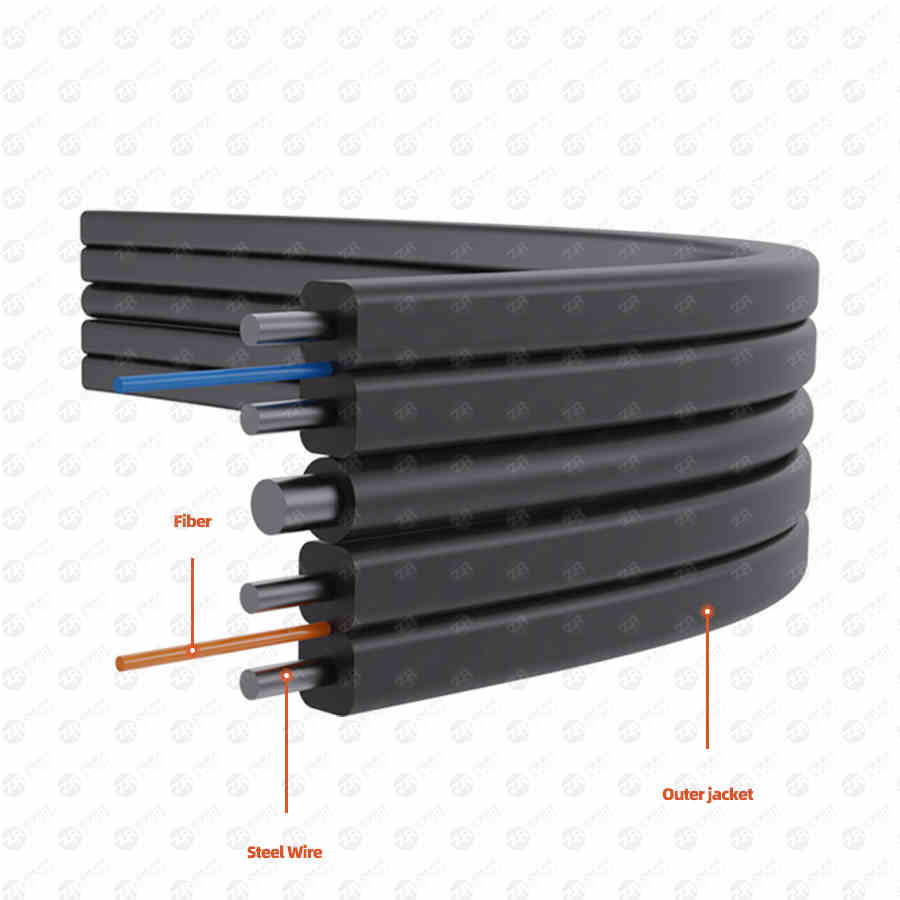# Calculation formula of cable consumption used in general wiring

2022-08-05
367

Some friends have always asked us how to determine the amount of cables in our integrated wiring. In fact, this is a common problem in construction. In this issue, we will learn about the calculation formulas in this area for your reference. There are mainly three types of formulas. .

1. Demand for RJ-45 headers and information modules

m: Indicates the total demand for RJ-45 connectors

n: indicates the total amount of information points

n*4*15%: Indicates the remaining surplus

Demand for information modules: m=n+n*3%

m: Indicates the total demand for information modules

n: indicates the total amount of information points

n*3%: Indicates the surplus

2. The amount of wire used for each floor

1. The amount of wire used on each floor:

C=[0.55*(L+S)+6]*n

L: The distance of the information point farthest from the management room on this floor

S: The distance from the information point closest to the management room on this floor

n: The total number of information points on this floor

0.55: Spare factor6: Termination tolerance

Of course, there is a second algorithm for the wire consumption of each floor, which is calculated according to the number of information sockets. This can be used flexibly in the project. You can see the formula below.

2. C=[0.55(F+N)+6]*m

C Line consumption per floor

F is the distance between the farthest information socket and the wiring room

N is the distance between the nearest information socket and the wiring room

m is the number of information sockets on each layer

3. The number of wire boxes

1. The longest line spacing + the shortest line spacing)/2=average

(average + 5 meters) * points = total length

Total length/305 (standard meters per box) + 2 boxes = total number of boxes

Remark:

The number of + can also be determined by yourself. If it is the first time, it is better to prepare more and not regret it later.

2. Each service requires a 4-pair unshielded twisted pair cable or a 2-core (62.5/125 micron multimode) optical cable;

The total number of horizontal cables in each communication room = (the number of work areas served by the communication room) * (the number of services provided by each work area)

Work area horizontal wiring calculation:

A: Distance to the nearest information point;

B: the distance of the farthest information point;

C: The number of information points in the work area on each floor

Cable length required for each layer=(A+B)/2*1.1*C

Sum of cable lengths of each layer = cable length of each layer * building height

The total number of cable boxes required = the sum of the cable lengths of each layer / 305 meters

Among them: 1.1 coefficient is loss, floor height is floor height, if the horizontal trunking goes to the ceiling, it must be calculated; if it is a raised floor, it can be ignored;

These three types of formulas are commonly used in wiring projects.

Previous OneOptical cable line failure
All
Next One24 core fiber optic distribution frame
Phone/Wechat008615211074652
Message